# 3.3b Union of Sets

3.3b Union of Sets

1. The union of set and set B, denoted by υ is the set consisting of all elements in set A or set B or both the sets.
The Venn diagram of υ B is illustrated as below: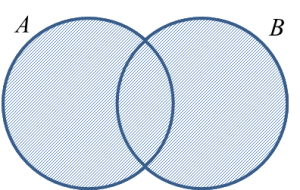2.
The union of set A, set B and set C, denoted by υ υ C is the set
consisting of all elements in set A, set B or set C or all the three sets.
The Venn diagram of υ υ C is illustrated as below: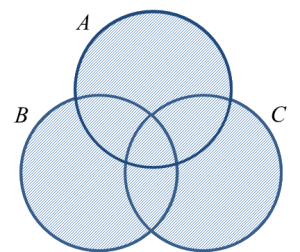Example 1:
The Venn diagram below shows the number of elements in the universal set, ξ, set P, set Q and R.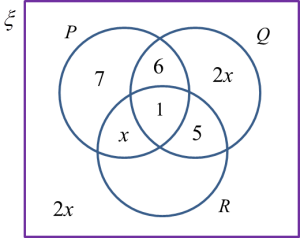Given n(Q) = n(P υ R)’, find n(ξ).

Solution:
n(Q) = n (P υ R)’
2x + 6 + 1 + 5 = 2x + 2x
2x + 12 = 4x
2x = 12
= 6

n(ξ) = 2x + 2x + x + 7 + 6 + 1 + 5
= 5x+ 19
= 5(6) + 19
= 30 + 19
= 49

Example 2:
Diagram below is a Venn diagram showing the universal set, ξ = {Form 3 students}, set = {Students who play piano} and set = {Students who play violin}.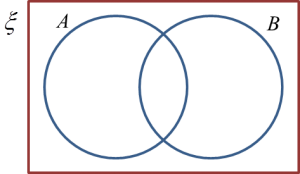Given n(ξ) = 60, n(A) = 25, n(B) = 12 and n(AB) = 8, find the number of students who do not play the two instruments.

Solution:
The students who do not play the two instruments are represented by the shaded region, (A υ B)’.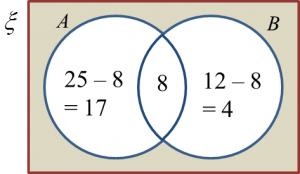Number of students who do not play the two instruments
= n (A υ B)’
= 60 – 17 – 8 – 4
= 31